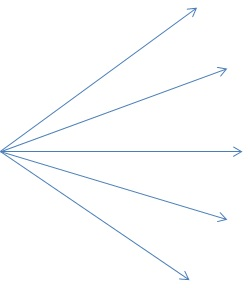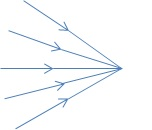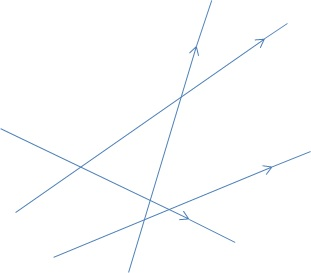Optics is the science of light. Visible light is described as the electromagnetic wave in the sensitivity range of the human eye. Light in the frequency range of 4*1014 to 8*1014 Hz is visible to the human eye.
Are you more of a visual learner? Check out our online video lectures and start your physics course now for free!Image: “Die waagerechten Linien sind exakt parallel.” by Fibonacci. License: CC BY-SA 3.0

Light sources and light propagation

Bodies that emit light, such as the sun, fixed stars, flames or a light bulb, are called self-luminous objects or light sources. A distinction is made between:

• Artificial light sources (light bulb, arc lamp)
• Natural light sources (sun, fixed stars)

The light that is emitted into the surrounding space, propagates straight in this space, as long as it encounters no obstacle. Light beams are used for the presentation of light. This is an imaginary straight line along which the light is thought to propagate. Light beams do not exist in reality, because the light emanating from a light source fans out in so called bundles of light. The boundary lines of light beams are illustrated by boundary rays or marginal rays. The proof of the linear propagation of light is shadowing.

 Type Definition Appearance divergent Rays emanating radially from a mutual point are divergent. They form a divergent light beam. The cross-section of the light beam increases.convergent Rays converging at a mutual point are convergent. They form a convergent light beam. The cross-section of the light beam decreases.parallel Rays extending always equally spaced are parallel. The beam cross-section remains the same.diffuse Rays, which have neither a common starting point nor a common destination, are diffuse. Cross-sections are not determinable.Reflection of light

Things will only be visible to us when light emanating from them reaches our eye. Illuminated bodies reflect light from their surface into our eyes.  There are two types of reflection: regular reflection and diffuse reflection.Regular reflection

Smooth surfaces produce a regular reflection of light. In such case, the angle of incidence is equal to the angle of reflection.

Diffuse reflection (Scattering)

Rough surfaces scatter light in all directions and thereby produce a diffuse reflection. Such a reflection is visible from any side. Examples are room walls, the moon, and the clouds. The indirect lighting produced by diffuse reflection has reduced glare and reduced shadows.

Reflection in mirrors

Plane mirrors

A plane mirror is common in everyday life. In it objects and images are symmetrical with respect to the mirror plane. The mirror image is virtual and appears as far behind the mirror as the object is in front of it.

Curved mirrors

Mirrors with curved surfaces are also common.  An example is the headlights of a car. Let’s consider the following types of curved mirrors:

Concave mirrors

The mirror surface of concave mirrors correlates with a part of the inside of a spherical surface. They are curved inward. The line from the sphere’s center to the mirror’s center is called the optical axis.

If a parallel beam of light reaches a curved mirror then the rays unite at the focal point. The distance of the focal point of a mirror’s center is called the focal distance. The focal distance is interpreted as half of the radius of the curvature by the viewer.

f = r / 2

An incident beam parallel to the axis passes, after reflection, through the focal point. The parallel ray becomes the focal ray.

An incident beam through the focal point passes, after reflection, parallel to the axis. The focal ray becomes the parallel ray.

An incident beam through the sphere’s center passes, after reflection, back into itself. The center ray remains unchanged.

Convex mirrors

With convex mirrors, the mirror surface correlates with part of a sphere’s surface. The outwardly curved portion of the spherical surface reflects light. The images of the convex mirror are always upright and down-scaled.

The closer the object is to the mirror, the more the image size approaches the object’s size. An incident parallel beam is reflected as if it comes from a point f behind the mirror—the so-called virtual focal point. An incident divergent beam is divergent after reflection, and its opening angle is greater than the one of the incident beam. The peripheral rays can be constructed with the help of the law of reflection.

The relationship between object distance, image distance and focal lengths for concave mirrors is also valid here. However, the focal length and the image distance have to be with a negative sign, since they are behind the mirror.

Parabolic Mirror

With parabolic mirrors, the reflecting surface resembles the section of a parabola. All incident light beams parallel to the axis, and even off-axis, run through the focal point after reflection. Parabolic mirrors are used to reflect the light in car headlights.

Absorption of light

White walls reflect light better than grey or black. With black walls, almost no reflection of the incident light occurs. The part of the light which is not reflected by a surface is absorbed. Absorbed light radiation is usually converted into heat energy.

Refraction of light

When a light beam crosses the boundary of two transparent media with different light velocities some part of the light can be reflected while another part is refracted. During the passage from an optically-thinner medium to an optically-denser medium, a light beam is refracted towards the perpendicular. If the light beam travels from the optically-denser medium to the optically-thinner medium, then it is refracted away from the perpendicular. A ray traveling perpendicular to the boundary between these two media is not refracted and does not change direction.

Incident ray refracted ray and the normal are in the same plane.

The refractive ratio depends only on the nature of the two media. The angle of incidence of the light beam does not affect the refraction ratio. According to Snell’s law it is possible to construct the ray path between two media when the refraction index is known.

Total internal reflection

When light travels from an optically-thicker medium to the boundary with an angle of incidence greater than the critical angle then the light is completely reflected. The critical angle is reached when the angle of reflection is 90°.  An example of this is refraction at the critical angle when light crosses from water to air.

Example: The refraction at the critical angle when crossing from water to air:Optical lenses

Translucent bodies that are bounded by curved surfaces, usually spherical surfaces, are called optical lenses. The connection line of the center points M and M’ of the curved surface forms the optical axis of the lens. The points of intersection S1 and S2 of the two lens surfaces and the optical axis are called the vertex of the lens. The midpoint of the segment S1S2 is called the optical midpoint 0. The plane perpendicular to the optical axis through 0 is called the plane of the lens or the plane of refraction.

Rays through the focal point of the lens are called focal rays.

Rays through the optical midpoint are called midpoint rays.

Rays entering parallel to the optical axis are called parallel rays.

Types of lenses

The most common lenses are spherical. They are translucent and bounded by two spherical surfaces. The two types of spherical lenses are convex and concave.

Convex lenses

Convex lenses are thicker in the middle than on the edges. The way they refract light converges parallel or moderately divergent light beams so that the rays meet at a point.

It is possible to converge divergent beam bundles with converging lenses if the divergence is not too large.

Concave lenses

Concave lenses are thinner in the middle than on the edges. The way they refract light diverges the incident beam. An incident light beam parallel to the optical axis is refracted as if the individual rays are from a focal point in front of the lens. The distance of this point is the focal length (also known as the dispersal distance).

An incident light beam parallel to the optical axis is refracted as if the individual rays are from a focal point F in front of the lens. The distance of this pint is the focal length f, which is negative and is also known as dispersal distance.

Optical instruments

The human eye is essentially an optical instrument.

Almost spherical, the eye can be rotated by six muscles acting on it from all sides. The sclera (1) forms the outer shell and is continuous with the cornea (5). The iris (6) contains a small hole in the middle. The light rays penetrate into the interior of the eye through the pupil (7).

The refracting system of the eye consists of the cornea (5) and the lens (11). They function approximately like a convex lens refracting light onto the retina (13). The images of observed objects are real inverted images on the retina. A normal eye can see near objects as sharply as distant ones. This is accomplished by the eye’s capability to change its curvature and focal length, and thus its refracting power. This adaptability is called accommodation.

The microscope

A microscope consists of two lenses—the prime lens and the ocular. The prime lens is the one facing the observed object whereas the ocular (otherwise called an eyepiece) is in front of the eye.

The prime lens can be replaced with lenses of other focal lengths, and the object distance can be adjusted.

Using a microscope we see enlarged, inverted virtual images of the observed object. The total magnification of the microscope v is the product of the magnification of the object vand the magnification of the eyepiece vg.

v = va * vg

Possible exam question regarding optics

The answers are below the references.

1. Which of the following wavelength ranges corresponds most closely to the range of visible light?

1. 100 – 400 nm
2. 200 – 500 nm
3. 300 – 600 nm
4. 400 – 700 nm
5. 500 – 900 nm

2. Which statement is not true?

During the transition of monochromatic light from an optically-denser to an optically-thinner medium…

1. … the speed of light is decreased.
2. … the frequency of the light remains unchanged.
3. … the wavelength of light is increased.
4. … the light is refracted away from the normal.
5. … total reflection can occur.

3. During the transition of an obliquely incident light beam from the optically-thinner medium to the optically-denser medium, the beam is refracted towards the normal because the speed of propagation of light in the optical-thinner medium is smaller than in the optically-denser medium.

1. Statement 1: true, Statement 2: wrong, Combination: true
2. Statement 1: true, Statement 2: true, Combination: wrong
3. Statement 1: true, Statement 2: wrong, Combination: –
4. Statement 1: wrong, Statement 2: true, Combination: –
5. Statement 1: wrong, Statement 2: wrong, Combination: –(Votes: 3, average: 5.00)Loading...